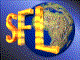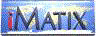| iMatix home page | << | < | > | >>SFLVersion 2.11

### descr2strt

```#include "sflstr.h"
char **
descr2strt (
const DESCR *descr)
```

#### Synopsis

Takes a descriptor prepared by strt2descr() and returns an array of strings pointers, terminated in a null pointer. The array is allocated using the mem_alloc() function. Each string is individually allocated. Thus, to free the string table you must call mem_free() for each entry in the table, except the last one, and then for the table. You can also call strtfree() to destroy the table in a single operation. Returns NULL if there was insufficient memory to allocate the table of strings.

#### Source Code - (sflstr.c)

```{
char
**table;
int
string_count,
string_nbr;                     /*  Index into string table          */
char
*descr_ptr;                     /*  Pointer into block               */

ASSERT (descr);

/*  Count the number of strings in the table                             */
descr_ptr = (char *) descr-> data;
string_count = 0;
while (*descr_ptr)                  /*  Loop until we hit null string    */
{
string_count++;
descr_ptr += strlen (descr_ptr) + 1;
}

/*  Allocate a table and fill it with the strings                        */
table = mem_alloc ((string_count + 1) * sizeof (char *));
if (table)
{
descr_ptr = (char *) descr-> data;
for (string_nbr = 0; string_nbr < string_count; string_nbr++)
{
table [string_nbr] = mem_strdup (descr_ptr);
descr_ptr += strlen (descr_ptr) + 1;
}
table [string_count] = NULL;    /*  Store final null pointer         */
}
return (table);
}
```

 | << | < | > | >>Copyright © 1996-2000 iMatix Corporation